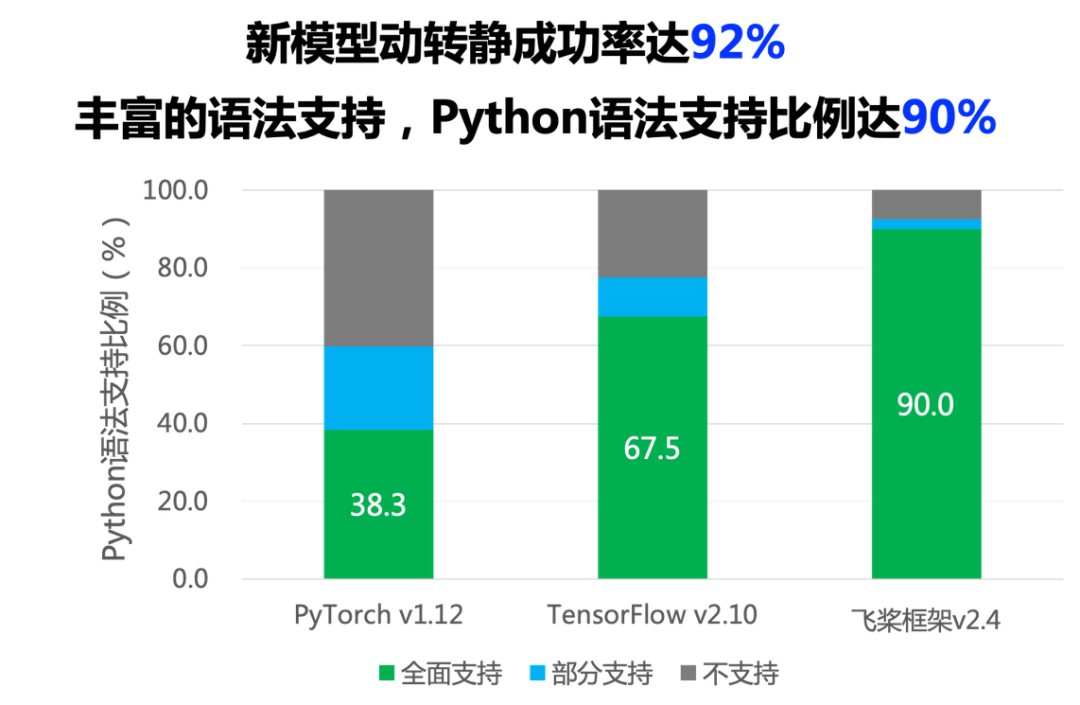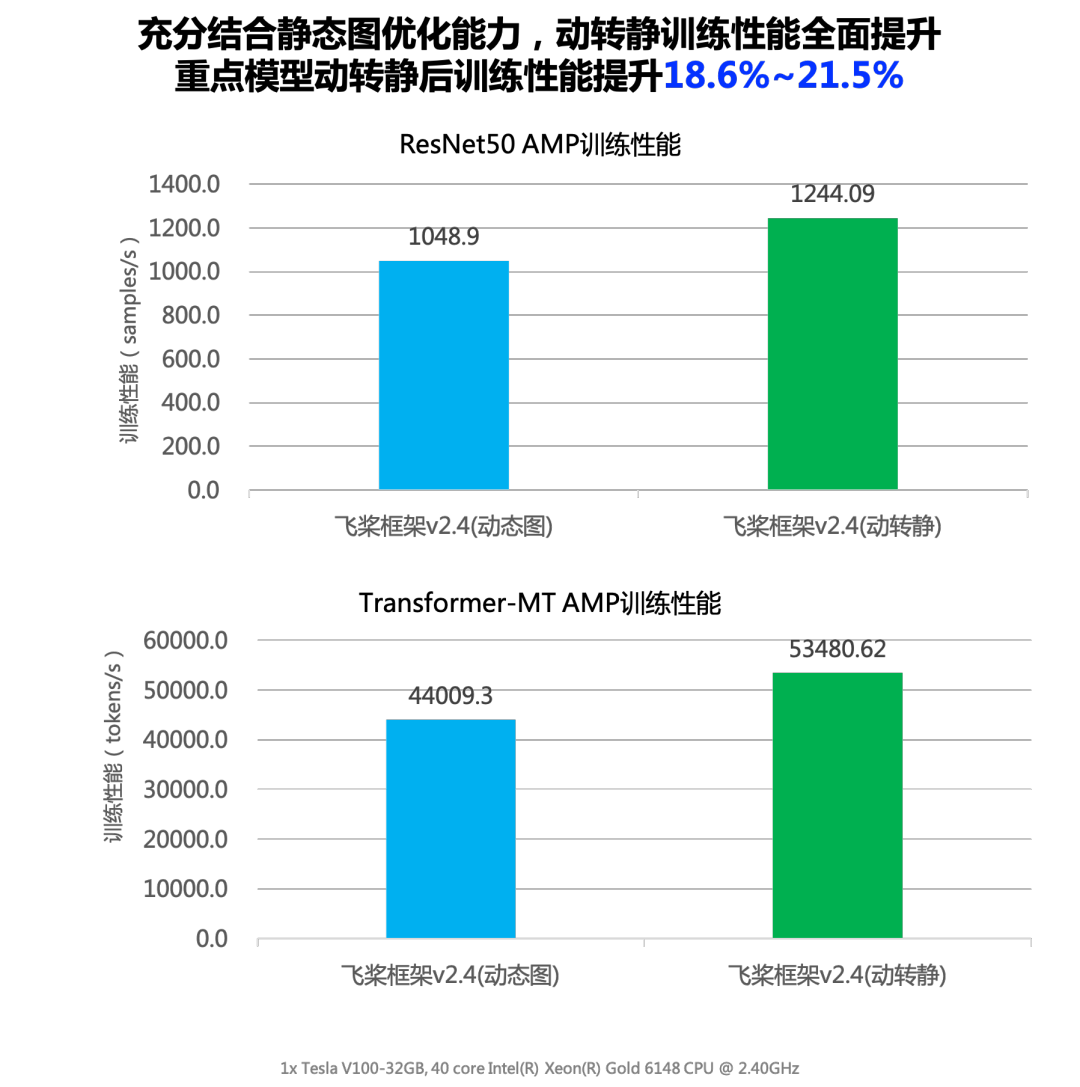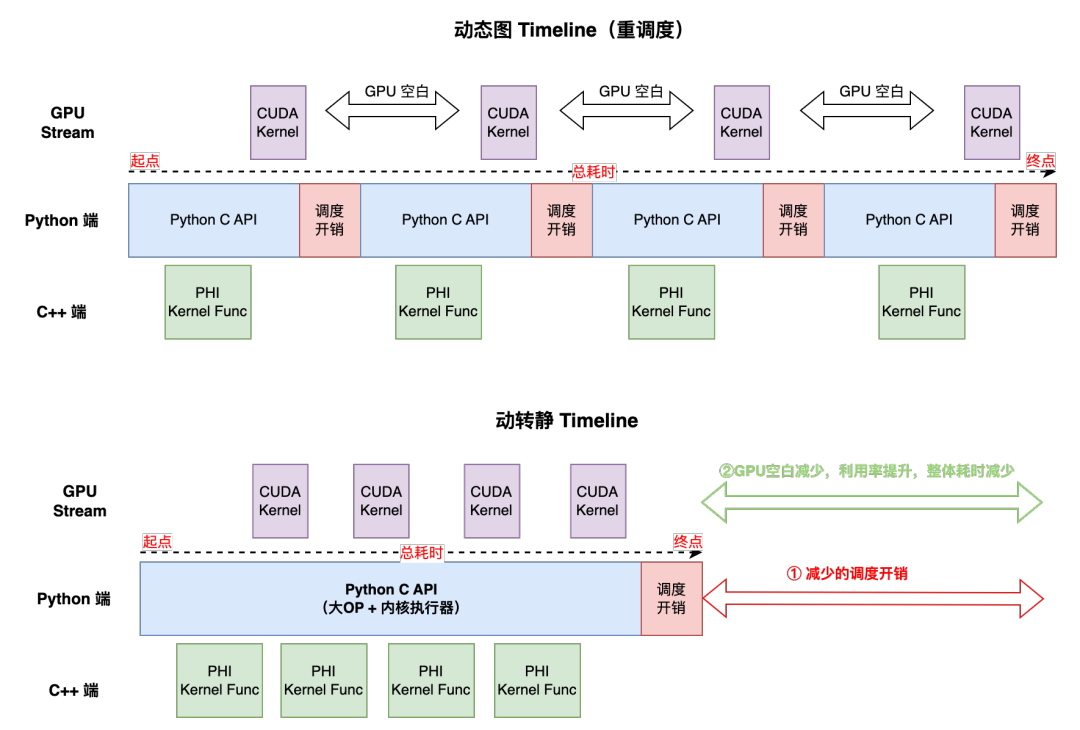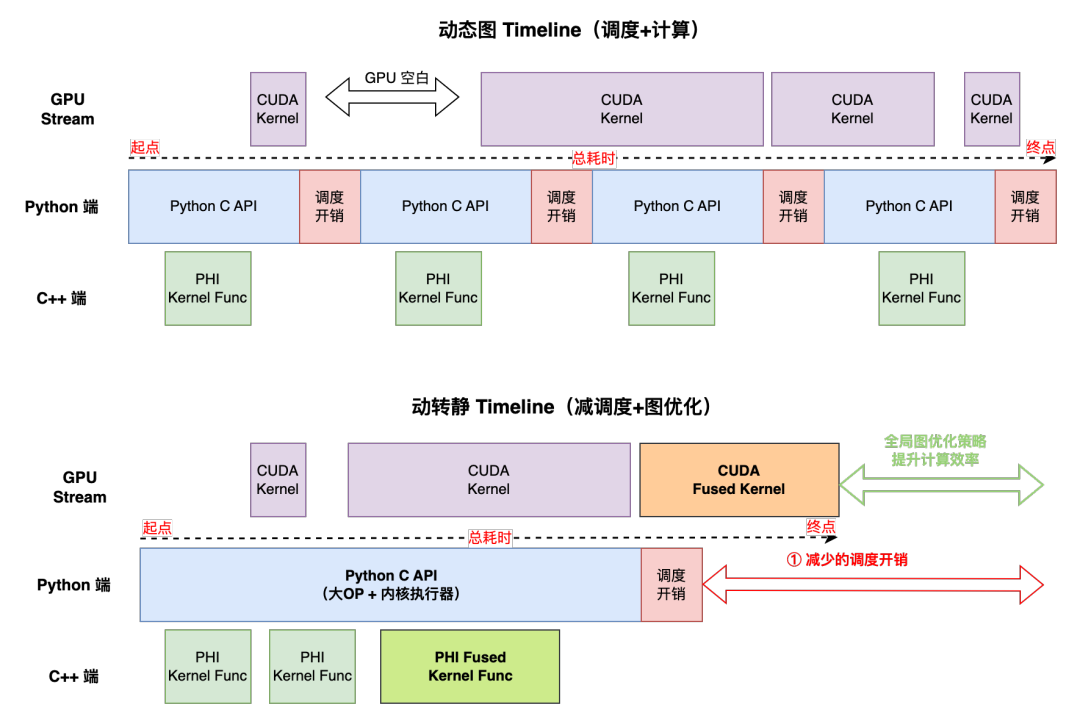# 動轉靜兩大升級！一鍵轉靜成功率領先，重點模型訓練提速18%+

• 動轉靜成功率明顯提升，一鍵轉換成功率達到92.1%。

• 轉靜訓練加速效果明顯，重點模型訓練可提速18%+。

# 一鍵轉靜成功率明顯提升

## API動靜行為統一

#### Einsum 升級``````import paddle

def __init__(self):
super(SimpleNet, self).__init__()

def forward(self, x):
out = self.linear(x)
out = out + 1
return out

net = SimpleNet()
train(net)  # 此處略去了訓練過程

# step 2: 切換到 eval() 模式
net.eval()
# step 3: 呼叫 jit.save 介面

```simple_net.pdiparams        // 存放模型中所有的權重資料
simple_net.pdmodel          // 存放模型的網路結構
simple_net.pdiparams.info   // 存放額外的其他資訊
```

• 新增裝飾器

• 切換 eval 模式

• 呼叫 save 介面

# 動轉靜訓練加速效果明顯## 場景一：重排程模型

• PU 利用率較低（可通過watch -n 1 nvidia-smi命令檢視）。

• 常見於NLP 領域或AMP/FP16 任務。

• 訓練效能瓶頸點主要是Host端排程開銷。• 一個Batch的訓練耗時取決於 Host 端總耗時。

• 動態圖每個Python API在執行時，都會產生一次Python 和C++互動，會產生較大的排程開銷。

• 動轉靜之後，整體上切分為執行前向和反向的兩個Python C API，故減少了很多個API間的排程開銷。

• 動轉靜核心執行器也經過了極致的優化（如Instruction快取等），Kernel launch效率也會比純動態圖模式要高。

```import paddle

def __init__(self):
super(SimpleNet, self).__init__()

def forward(self, x):
out = self.linear(x)
out = out + 1
return out

# create network
net = SimpleNet()

out = net(x)
loss.backward()
opt.step()

```

## 場景二：排程+計算共存模型

• GPU 利用率波動比較大（可通過watch -n 1 nvidia-smi命令檢視）。

• 訓練效能瓶頸點同時受區域性排程和區域性 Kernel 計算效率影響。• 一個Batch的訓練耗時取決於 Max(Host端，GPU端)。

• 動轉靜降低了Python C API 排程開銷，收益點大多在Batch前半部分，後半部分可能會被overlap，排程方面的收益會打折扣。

• 動轉靜可藉助全域性圖優化技術，通過運算元融合等技術提升模型訓練的吞吐。

```import numpy as np

def get_build_strategy():
# 運算元融合策略
return build_strategy

# 此處省略了模型定義
@to_static(build_strategy=get_build_strategy()) # 方式一
def forward(self, image):
# 省略前向程式碼

# create network
net = ResNet()
# 藉助 build_strategy 引數自定義開啟「全域性圖優化」策略
net = paddle.jit.to_static(net, build_strategy=get_build_strategy()) # 方式二

out = layer(image)
loss.backward()
opt.step()

```

 動轉靜使用樣例

 動轉靜訓練圖優化策略